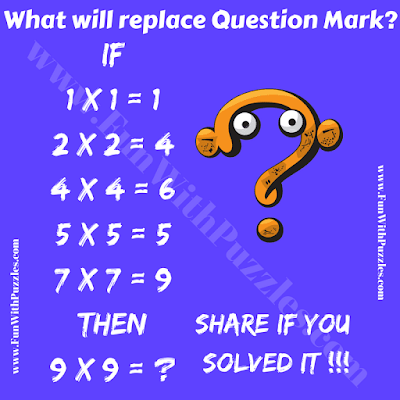Here is a very interesting Logical and Mathematical puzzle that will not only test your Mathematical skills but also your logical skills. In this Mathematics puzzle, there are some interesting number equations. These number equations are not correct Mathematically but there is a logical pattern in these equations which is followed by each of these given equations. Can you solve this Logic Math Puzzle by finding the hidden logical pattern in the given number equations? If you are able to crack the code, then find the value of the missing number to solve this Logic Math Puzzle?Logical and Mathematical Number Puzzle for Teens

The answer to this "Mathematics Puzzle", can be viewed by clicking on the button. Please do give your best try before looking at the answer.

Unknown said...

1

Manha said...

Iam manha from ulapane